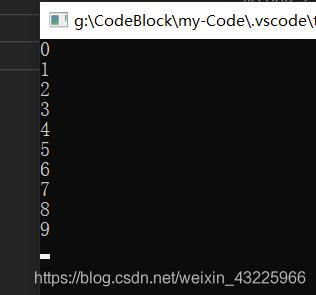# C语言实现简单的定时器

更新时间：2020年10月29日 12:54:59   作者：研究猿小刘

1.代码分析2.代码

```#include <stdio.h>
#include <time.h>
#include <conio.h>

#ifndef CLOCKS_PER_SEC
#define CLOCKS_PER_SEC 1000
#endif

int main( void )
{
clock_t start;
long count = 1;
start = clock();
while(1)
{
if((clock() - start) == CLOCKS_PER_SEC)
{
printf("%ld\n",count++);
start = clock();
//break;
}
}
getch();
}```

3. 代码抽象出一个定时器函数 void timer（long time）

```void timer(long time){

clock_t start;
long count = 1;
start = clock();
while(1)
{
if((clock() - start) != (time*CLOCKS_PER_SEC))
{
//时间没有到，啥也不做，空循环
}else {
//时间到了退出循环
// printf("%s","hello");
break;
}

}
}```

```#include <stdio.h>
#include <time.h>
#include <conio.h>

#ifndef CLOCKS_PER_SEC
#define CLOCKS_PER_SEC 1000
#endif
/**
* time 的单位为s
*/
void timer(long time){

clock_t start;
long count = 1;
start = clock();
while(1)
{
if((clock() - start) != (time*CLOCKS_PER_SEC))
{
//时间没有到，啥也不做，空循环
}else {
//时间到了退出循环
// printf("%s","hello");
break;
}

}
}
int main( void )
{

for(int i=0;i<10;i++){
timer(1);
printf("%d\n",i);
}
getch();
}```